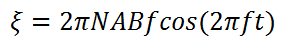# EMF induced in Electrical Generator calculator

This CalcTown claculator calculates the emf induced in an electrical generator, following Lenz's Law.

*Please note that the formula is valid only for sinusoidal magnetic fields and the output is the amplitude of the resulting field, which is of the form given in equation.

#### EMF induced in Electrical Generator calculator

m2
Tesla